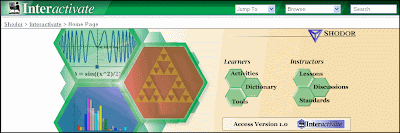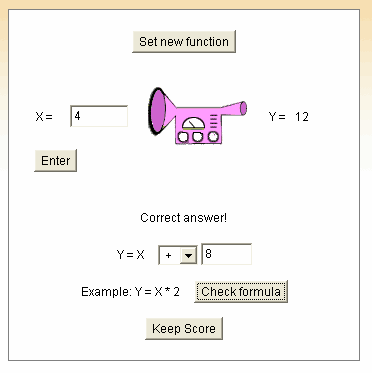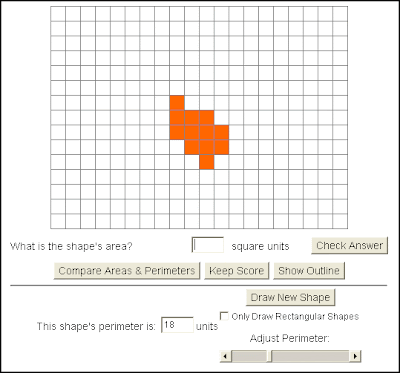I stumbled across a nice website last week called Interactivate with 140 (or so) interactive Java-based activities for algebra, geometry, probability, statistics, etc. Many of the activities are modifications of other activities, but still there are at least 30 unique game-based activities here to help your students learn.I particularly liked “Algebra Four” (a play on the game Connect Four). I am teaching my algebra students all about solving equations right now and this would give them some good practice. The student can choose the level of difficulty (one-step, two-step, distributive, etc.). So conceivably, a student could first play at the one-step level, then the two-step level, then add the distributive property, and work their way up. This is a two-player game, which is really the only drawback, as a student at home would have to play against themself (or convince someone else to play an algebra game with them… hmm… unlikely). I do like the timer, which would encourage the student to get faster at solving equations. And if a student doesn’t want to play against the timer, it could just be set for a high time.

Another nice game here is the “function machine” like we’ve seen in textbooks, only this one is really a machine where you (or the student) inputs values, and it (the machine) processes the values and outputs them. The “game” here is to guess the function. I could see using this one in a classroom when we talk about function notation, and writing out the function notation process of each “guess” and “answer” to the side of the projected machine. A small improvement on this game would be to show the function notation to the side and then run a version that would let the all-knowing instructor input “x” at the end of the game, to show that if you input “x” or “a” into the function notation, the function notation shows you exactly what happens during the processing.One last gem from this site is Area Explorer, which shows the student a graph of connected, shaded squares, and asks the student to find the area and perimeter. What I like is that it emphasizes the underlying principle behind area and perimeter (area is counting unit squares in the interior of the figure and perimeter is counting unit lines on the perimeter of the figure). Our students today seem to often miss this concept alltogether and just want to boil everything down to a formula, so I think this would be a great activity to have students try on their own.All in all… kudos to this site for creating some really nice interactive materials!

Possibly Related Posts: